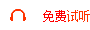# 2021年成考专升本《高数二》预习试题及答案三

1[.单选题]下列命题正确的是()。

A.函数f(x)的导数不存在的点，一定不是f(x)的极值点

B.若x0为函数f(x)的驻点，则x0必为f(x)的极值点

C.若函数f(x)在点x0处有极值，且f'(x0)存在，则必有f'(x0)=0

D.若函数f(x)在点x0处连续，则f'(x0)一定存在

[答案]C

[解析]根据函数在点x0处取极值的必要条件的定理，可知选项C是正确的。

2[.单选题]函数f(x)的导函数f'(x)的图像，则在(-∞，+∞)内f(x)的单调递增区间是()。

A.(-∞，0)

B.(-∞，1)

C.(0，+∞)

D.(1，+∞)

[答案]B

[解析]因为x在(-∞，1)上，f'(x)>0，f(x)单调增加，故选B。

3[.单选题]若f'(x)<0(a0，则在(a，b)内必有()。

A.f(x)>0

B.f(x)<0

C.f(x)=0

D.f(x)符号不定

[答案]A

[解析]因为f'(x)<0x∈(a，b)，所以f(x)单调减少x∈(a，b)。又f(b)>0，所以f(x)>0x∈(a，b)。

4[.单选题]设函数f(x)在x=1处可导，且f'=0，若f">0，则f是()。

A.极大值

B.极小值

C.不是极值

D.是拐点

[答案]B

[解析]由极值的第二充分条件可知，应选B。

5[.单选题]设u=u(x)，v=v(x)是可微的函数，则有d(uv)=()。

A.udu+vdv

B.u'dv+v'du

C.udv+vdu

D.udv-vdu

[答案]C

6[.单选题]设函数y=sin(x2-1)，则dy等于()。

A.cos(x2-1)dx

B.-cos(x2-1)dx

C.2xcos(x2-1)dx

D.-2xcos(x2-1)dx

[答案]C

[解析]dy=y'dx=cos(x2-1)(x2-1)'dx=2xcos(x2-1)dx

7[.单选题]设函数ƒ(x)=exlnx，则ƒ'=()。

A.0

B.1

C.e

D.2e

[答案]C

8[.单选题]d(sin2x)=()。

A.2cos2xdx

B.cos2xdx

C.-2cos2xdx

D.-cos2xdx

[答案]A

9[.单选题]设函数y=e2x+5，则y'=( ).

A.e2x

B.2e2x

C.2e2x+5

D.2ex+5

[答案]B

>>学课在线网课试听.报名        >>>>录播课程
 课程名称 课程免费试听 课程名称 课程免费试听 课程名称 课程免费试听 初级会计师一级建造师执业药师中级会计师二级建造师护士资格证注册会计师一级造价工程师健康管理师经济师考试监理工程师考研辅导课银行从业安全工程师自考辅导课基金从业一级消防工程师心理咨询师企业人力资源管理师法律职业资格考试公共营养师社会工作者消防设施操作员保育员教师资格证成人高考育婴师>>长期招聘兼职招生代理人员，项目合作，团报优惠咨询，有意者请联系我们 >>咨询：13312524700（可加微信）。

## 【云南考试培训报名咨询】微信扫一扫关注我们